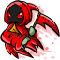Discussion about math, puzzles, games and fun.   Useful symbols: ÷ × ½ √ ∞ ≠ ≤ ≥ ≈ ⇒ ± ∈ Δ θ ∴ ∑ ∫  π  -¹ ² ³ °

You are not logged in.

## #1 2007-10-16 09:10:06

TomHawk
Member
Registered: 2007-10-16
Posts: 4

### ln A=ln h - ln(1-h); Solve for h

Need some help with this problem.  I have the answer, just don't understand how you get this answer.

Answer: h = A / (A + 1)

I would appreciate it if someone could show me the mechanics of this equation.

Thanks.

Offline

## #2 2007-10-24 14:51:14

TheDude
Member
Registered: 2007-10-23
Posts: 361

### Re: ln A=ln h - ln(1-h); Solve for h

Better late than never:

This isn't really a coding problem, but here's the method anyway.  From the original equation we know a couple facts about A and h.  First, we know that A > 0 (strictly greater than) because ln is undefined for values of A <= 0.  For the same reason we know that h > 0.  However, we also know that 1 - h > 0, which implies h < 1 (again, strictly).   This gives us A > 0 and 0 < h < 1.

Now, we first simplify the equation by combining the logs on the right side.  ln(a) - ln(b) = ln(a / b) is a logarithmic identity, so we get ln(A) = ln(h / (1 - h) ).

Now, since the exponential function is continuous over the real numbers we can take the exponential of both sides to remove the logs and get A = h / (1 - h).

From here on out it's just algebra, but for the sake of completeness I'll go step by step.  Since we know both A and h are strictly greater than 0 we can take the reciprocal of both sides to give us 1 / A = (1 - h) / h.  We then split up the fraction on the right side to get 1 / A = (1 / h) - 1.  Add 1 to give us 1 + (1 / A) = 1 / h --------> (A + 1) / A = 1 / h.  Again, A + 1 > 0, so take the reciprocal again to give us A / (A + 1) = h   QED.

Last edited by TheDude (2007-10-24 14:52:19)

Wrap it in bacon

Offline

## #3 2007-10-24 22:49:49

Zach
MemberRegistered: 2005-03-23
Posts: 2,075

### Re: ln A=ln h - ln(1-h); Solve for h

[ M-m-m-m-MOVED! ]

Boy let me tell you what:
I bet you didn't know it, but I'm a fiddle player too.
And if you'd care to take a dare, I'll make a bet with you.

Offline